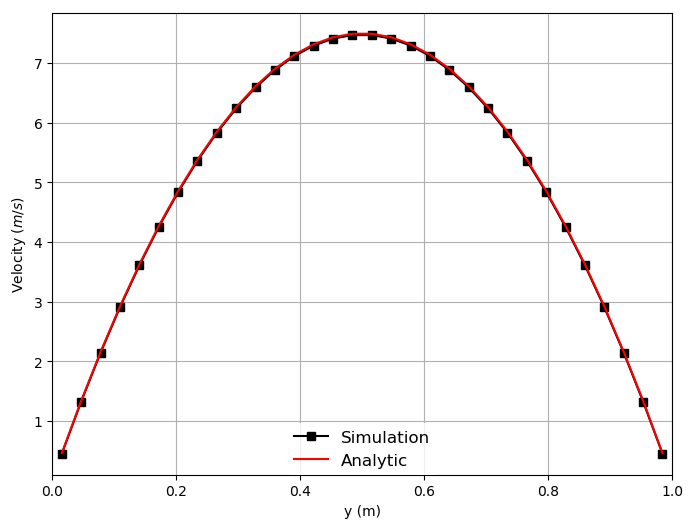# Poisueille flow in a channel 2D

Navigate: ← Test case channel 2D

# Poisueille flow in a channel 2D using hrrBGK collision scheme

In this example, we will investigate the Poiseuille flow in a plain 2D channel using the hybrid recursive regularized bgk (hrrBGK) collision scheme with a fourth order regularization.

This collision scheme is called as below in the identify table. relaxation = 'hrr_bgk'

The fluid table in musubi.lua should include the following --! [Fluid] fluid = { kinematic_viscosity = nu_phy, hrr_sigma = 0.99 -- default = 0.98 } --! [Fluid]

The sigma has a default value of 0.98 which can be overwritten from input. This represents a blending coefficient, therefore must be greater equal than 0.0 and lower equal than 1.0. A value of 0.00 reverts the HRR to the PRR scheme. A value of 1.0 reverts the HRR into the RR scheme. Any value in between is a blended scheme. For non dissipative runs, try to stay closer to 0.98. The RR scheme dissipates less than the PRR scheme.

A detailed description of test case can be found in the parent directory Description of test case channel 2D.

## Post-processing

Here are the results from the simulation. To create them, double the number of elements in height (nHeight=32) in seeder.lua.

Velocity along the height of the channel:Pressure across the length of the channel:Wall shear stress along the height of the channel:To create these plots, run python plot_track.py to create the plots. Before running the plot script, open 'plot_track.py' and update path to Gleaner script in 'glrPath'. Download Gleaner script using hg clone https://geb.sts.nt.uni-siegen.de/hg/gleaner

Musubi was developed by University of Siegen